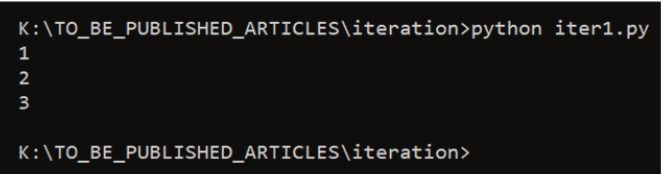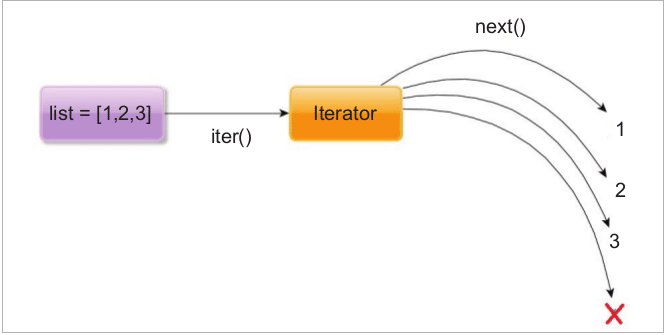# How are Iterable, Iterator and Generator Different?

1
1909Advertisement

The concepts — iterable, iterator and generator — can be pretty confusing to Python users. This article highlights the differences between the three advanced concepts and guides you on how they may be used effectively.

In Python programming, iterable, iterator and generator are the most confusing concepts because many cannot tell what differentiates them. For example, in the Python 2.7 dictionary data structure, we can use dict.items() as well as dict.iteritems() for iterating the dictionary. Both the methods work in the same way, but if we talk about memory and performance, there is a huge difference. dict.items() returns a list that is iterable and dict.iteritems() is a generator.

Containers

Containers are the data structures that hold data or values. They are easy to understand because you can think of them as real-life containers like boxes, trucks, houses, etc. In Python, programming containers are lists, dictionaries and tuples.

Advertisement

Iteration/iterable protocols

• Iter() creates an iterator. Iter() is called on the iterable to retrieve an iterator.
• Next() is called on the iterator to sequentially retrieve elements from iterable.
• When no more elements are available, next() will raise StopIteration.

Figure1 illustrates the relationship between iterator and iterable.Figure 1: Relation between iterator and iterable

Iterable

Iterable is an object that implements the __iter__ method. An iterable is any object (not necessarily a container) that can be a file pointer and return an iterator (with the purpose of returning all of its elements). A Python list object is iterable. Let’s check the built-in method of a list.

```>>> dir([])

[‘__add__’, ‘__class__’, ‘__contains__’, ‘__delattr__’, ‘__delitem__’, ‘__dir__’, ‘__doc__’, ‘__eq__’, ‘__format__’, ‘__ge__’, ‘__getattribute__’, ‘__getitem__’, ‘__gt__’, ‘__hash__’, ‘__iadd__’, ‘__imul__’, ‘__init__’, ‘__init_subclass__’, ‘__iter__’, ‘__le__’, ‘__len__’, ‘__lt__’, ‘__mul__’, ‘__ne__’, ‘__new__’, ‘__reduce__’, ‘__reduce_ex__’, ‘__repr__’, ‘__reversed__’, ‘__rmul__’, ‘__setattr__’, ‘__setitem__’, ‘__sizeof__’, ‘__str__’, ‘__subclasshook__’, ‘append’, ‘clear’, ‘copy’, ‘count’, ‘extend’, ‘index’, ‘insert’, ‘pop’, ‘remove’, ‘reverse’, ‘sort’]```

The __iter__ method is shown above. When we can do iter() then the iter() function calls the magic __iter__ method.

In order to receive the element one by one, and sequentially, the iterable is converted to an iterator first, and then the next() function is used to get the elements from the iterator. Internally, the next() function calls the magic method __next__.

Let us use the next function with list as an argument:

```>>> list1 = [1,2,3]

>>>

>>> next(list1)```

Traceback (most recent call last):

```File “<stdin>”, line 1, in <module>

TypeError: ‘list’ object is not an iterator

>>>```

You can see the error list is not an iterator. In order to use the next() function, make the list iterable. Let’s examine the following command:

```>>> dir(list_new)

['__class__', '__delattr__', '__dir__', '__doc__', '__eq__', '__format__', '__ge__', '__getattribute__', '__gt__', '__hash__', '__init__', '__init_subclass__', '__iter__', '__le__', '__length_hint__', '__lt__', '__ne__', '__new__', '__next__', '__reduce__', '__reduce_ex__', '__repr__', '__setattr__', '__setstate__', '__sizeof__', '__str__', '__subclasshook__']

>>>

>>> next(list_new)

1

>>> next(list_new)

2

>>> next(list_new)

3

>>> list_new

<list_iterator object at 0x000001B9A6A8A550>

>>>```

For more clarification, look at Figure 2. You can see the __next__ method is the built-in method of the iterator.

Let us write the code to get the list values by using the while loop with the help of the iteration protocol:

```list1 = [1,2,3]

list2 = iter(list1)

while True:

try:

print (next(list2))

except StopIteration as e:

break```

Check the output in Figure 3.

In the above code, an iterator is formed from the list by using the iter() function. The rest of the code is self-explanatory. The for loop first converts the iterable to an iterator. Let us examine the following piece of code:

```>>> import dis

>>> list1 = [1,2,3]

>>> exp1 = “for i in list1: print (i)”

>>> dis.dis(exp1)

1 0 SETUP_LOOP 20 (to 22)

2 LOAD_NAME 0 (list1)

4 GET_ITER

>> 6 FOR_ITER 12 (to 20)

8 STORE_NAME 1 (i)

10 LOAD_NAME 2 (print)

12 LOAD_NAME 1 (i)

14 CALL_FUNCTION 1

16 POP_TOP

18 JUMP_ABSOLUTE 6

>> 20 POP_BLOCK

>> 22 LOAD_CONST 0 (None)

24 RETURN_VALUE

>>>```

When you disassemble this Python code, you can see the explicit call to GET_ITER, which is similar to invoking iter(list1). The FOR_ITER is an instruction that will do the equivalent of calling next() repeatedly to get every element.Figure 2: List to iterator conversion

Iterator

An iterator is an object that implements the iterable protocol as it also responds to the __next__() method. To use iterable and iterator, there is a simple iterable protocol which is driven by two functions, iter() and next().

Let us create an example:

```>>> list1 = [1,2,3]

>>> list_new = iter(list1)

>>> list_new

<list_iterator object at 0x000001AA40A504A8>

at 0x000001AA40A504A8>

>>> list_new1 = iter(list_new)

>>> list_new1

<list_iterator object at 0x000001AA40A504A8>

>>>```

You will notice that both the objects refer to the same memory address. If you pass an iterator to iter then the __iter__ method returns the same object.

Let us create an iterator myrange that will work like xrange() of the Python 2.7 class MyRange():

```def __init__(self,a,b=0,c=1):

if b==0:

self.a = a

self.b = b

elif b!=0:

self.b=a

self.a = b

self.c = c

def __iter__(self):

return self

def __next__(self):

if self.b<self.a:

self.b= self.b+self.c

return self.b

else:

raise StopIteration

if __name__ == “__main__”:

a1 = MyRange(3)

print (next(a1))

print (next(a1))```

Let us look at the output:

```>>> from myrange1 import MyRange

>>>

>>> m1 = MyRange(0,10,2)

>>>

>>> for each in m1:

... print (each)

...

2

4

6

8

10```

Let us see another output.

```>>> m1 = MyRange(5)

>>> for each in m1:

... print (each)

...

1

2

3

4```

Let us list with it.

```>>> m1 = MyRange(5)

>>> list(m1)

[1, 2, 3, 4, 5]

>>>```

Now, you have probably understood the differences between iterable and iterator. Let us move on to the next topic.Figure 3: Output of the iter1.py program

Generator

A generator is a special kind of iterator. All generators are iterators but all iterators are not generators. Each time the yield statement is executed, the generator function generates a new value.

When a generator function gets called, then a generator object is returned without even beginning the execution of the generator function. When the next() function is called for the first time, the generator function starts executing until it reaches the yield statement. The yielded value is returned by the next() call.

Figure 4 shows that a generator or generator expression is always an iterator.

Now let us look at the code for a generator:

```def myrange(a):

i = 0

while i < a:

yield i

i = i+ 1```

Let us see the output:

```>>> from gene1 import myrange

>>> a = myrange(4)

>>> next(a)

0

>>> next(a)

1

>>> next(a)

2

>>> next(a)

3

>>> next(a)```

Traceback (most recent call last):

```File “<stdin>”, line 1, in <module>

StopIteration```

Generator expression

Let us understand the generator expression. The generator function is that in which the keyword yield appears in its body. The other type of generator is the generator expression, which is equivalent to a list comprehension. Its syntax is very similar to the list comprehension.

Let us look at the following series of examples.

```>>> import sys

>>> list1 = [2,3,4,5,6]

>>>

>>> list2 = [each*2 for each in list1]

>>>```

List2 contains values that are multiples of 2.Figure 4: The relationship between the generator, iterable, iterator and container

Let us look at the size of list3. You can see that the size of list2 is greater than list1. But the size of list3 is the same.

```>>> list2

[4, 6, 8, 10, 12]```

Let us look at the size of list1:

```>>> sys.getsizeof(list1)

104```

Let us examine the size of list2:

```>>> sys.getsizeof(list2)

128

>>> list3 = (each*2 for each in list1)

>>>

>>> next(list3)

4

>>> next(list3)

6

>>> next(list3)

8

>>> next(list3)

10

>>> next(list3)

12

>>> next(list3)```

Traceback (most recent call last):

```File “<stdin>”, line 1, in <module>

StopIteration

>>>```

Let us compare the memory consumption of a generator expression and a list comprehension.

```>>> import sys

>>> list1 = list(range(100000))

>>> list2 = [each*2 for each in list1]

>>> sys.getsizeof(list2)

824464

>>>

>>> list3 = (each*2 for each in list1)

>>> sys.getsizeof(list3)

120```

You can see there is a huge difference in memory consumption. Let us compare the performance. Look at the following code and output.

```import datetime

main_list = list(range(100000000))

t1 = datetime.datetime.now()

list1 = [each**2 for each in main_list]

for each in list1:

each

t2 = datetime.datetime.now()

print (t2-t1)```

I ran the code three times and checked the performance.

```K:\TO_BE_PUBLISHED_ARTICLES\iteration>python3 iter4.py

0:00:51.652933

K:\TO_BE_PUBLISHED_ARTICLES\iteration>python3 iter4.py

0:00:50.144020

K:\TO_BE_PUBLISHED_ARTICLES\iteration>python3 iter4.py

0:00:53.793114```

When the list comprehension is converted to the generator, look at the following code:

```import datetime

main_list = list(range(100000000))

t1 = datetime.datetime.now()

list1 = (each**2 for each in main_list)

for each in list1:

each

t2 = datetime.datetime.now()

print (t2-t1)```

The output is:

```K:\TO_BE_PUBLISHED_ARTICLES\iteration>python3 iter4.py

0:00:41.742375

K:\TO_BE_PUBLISHED_ARTICLES\iteration>python3 iter4.py

0:00:40.739899

K:\TO_BE_PUBLISHED_ARTICLES\iteration>python3 iter4.py

0:00:40.509761```

The above result concludes that the generator’s performance is better.

Advertisement
SHAREThe author, who is a CEH and ECSA, has completed a Masters in Engineering (ME) in computer science from Thapar University, Patiala. He has worked in IBM, Teramatrix and Sapient. He is the author of books Python penetration testing essential, Python: Penetration testing for developers and Learn Python in 7 days.

#### 1 COMMENT

1.Mike Maxwell

This is a bit confusing. Near the top of the article, there are lines like
dir(list_new)
next(list_new)
But list_new isn’t introduced until later in the article:
Let us create an example:
>>> list1 = [1,2,3]
>>> list_new = iter(list1)
I think the latter part should have appeared before the former, otherwise the reader is left wondering where this list_new came from.